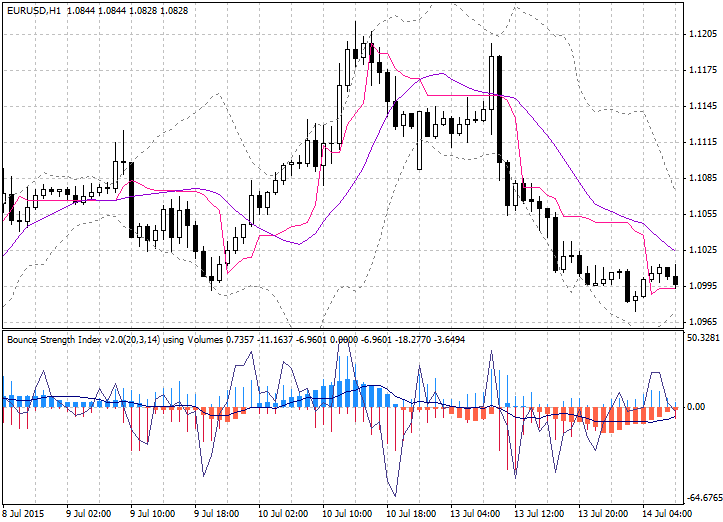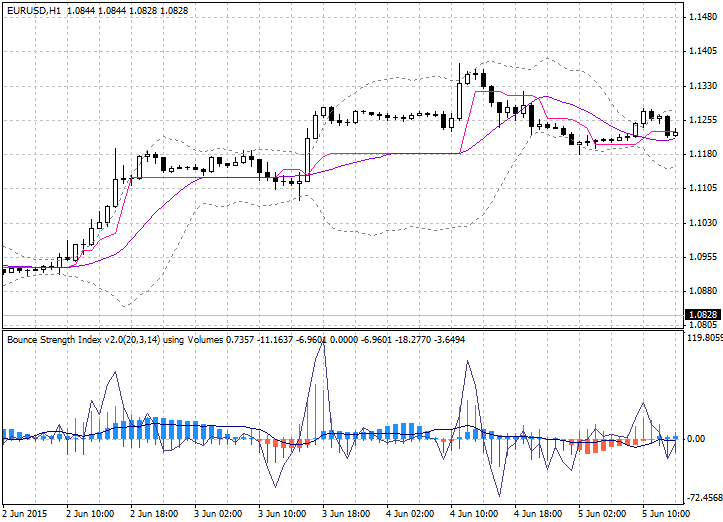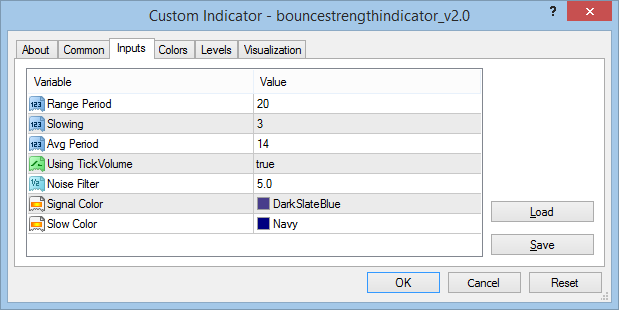Bounce Strength Indicator 2.0 with Tango Line

# Bounce Strength Indicator 2.0 with Tango Line

19397
0
SHAREThis indicator shows bounce strength, essential for breakout or retracement positions in any time frame.

I used the width of the range as a measure to measure (like a classical oscillator). This version does not use the width of the range. It uses the deviation from the center line instead. And the center line is the moving average of the Tango Line.

double ratio=0;

double sp=(high[k]-low[k]);

//— Not DownBar

if(!(close[k-1]-sp*0.2>close[k]))

{

ratio=-1*(low[k]/TangoMaBuffer[k])+2;

sumpos+=(close[k]-low[k])*ratio;

}

//— Not UpBar

if(!(close[k-1]+sp*0.2<close[k]))

{

ratio=-1*(high[k]/TangoMaBuffer[k])+2;

sumneg+=(high[k]-close[k])*ratio;

}

Display:

• Plus histogram — shows the bounce strength up from lows.
• Minus histogram — shows the bounce strength down from highs.
• Signal line — difference between plus/minus [ (plus – minus) * abs((plus-minus) / (plus+minus)) ].
• Blue box — uptrend strength (average of Signal line).
• Red box — downtrend strength (average of Signal line).
• Slow Line — average of Signal line (long span).

Chart window is Tango Line (version 1.1).Settings:

//— input parameters

input int InpRangePeriod=20; // Range Period

input int InpSlowing=3; // Slowing

input int InpAvgPeriod=14; // Avg Period

input bool InpUsingVolumeWeight=true; // Using TickVolume

input double InpReversalNoiseFilter=5; // Noise Filter

input color InpSigColor=DarkSlateBlue; // Signal Color

input color InpSlowColor=Navy; // Slow ColorBounce Strength Indicator 2.0 with Tango Line is a Metatrader 4 (MT4) indicator and the essence of the forex indicator is to transform the accumulated history data.

Bounce Strength Indicator 2.0 with Tango Line provides for an opportunity to detect various peculiarities and patterns in price dynamics which are invisible to the naked eye.

Based on this information, traders can assume further price movement and adjust their strategy accordingly.

How to install Bounce Strength Indicator 2.0 with Tango Line.mq4?

• Copy Bounce Strength Indicator 2.0 with Tango Line.mq4 to your Metatrader Directory / experts / indicators /
• Select Chart and Timeframe where you want to test your indicator
• Right click on Bounce Strength Indicator 2.0 with Tango Line.mq4
• Attach to a chart
• Modify settings or press ok
• Indicator Bounce Strength Indicator 2.0 with Tango Line.mq4 is available on your Chart

How to remove Bounce Strength Indicator 2.0 with Tango Line.mq4 from your Metatrader 4 Chart?

• Select the Chart where is the Indicator running in your Metatrader Client
• Right click into the Chart
• “Indicators list”
• Select the Indicator and delete